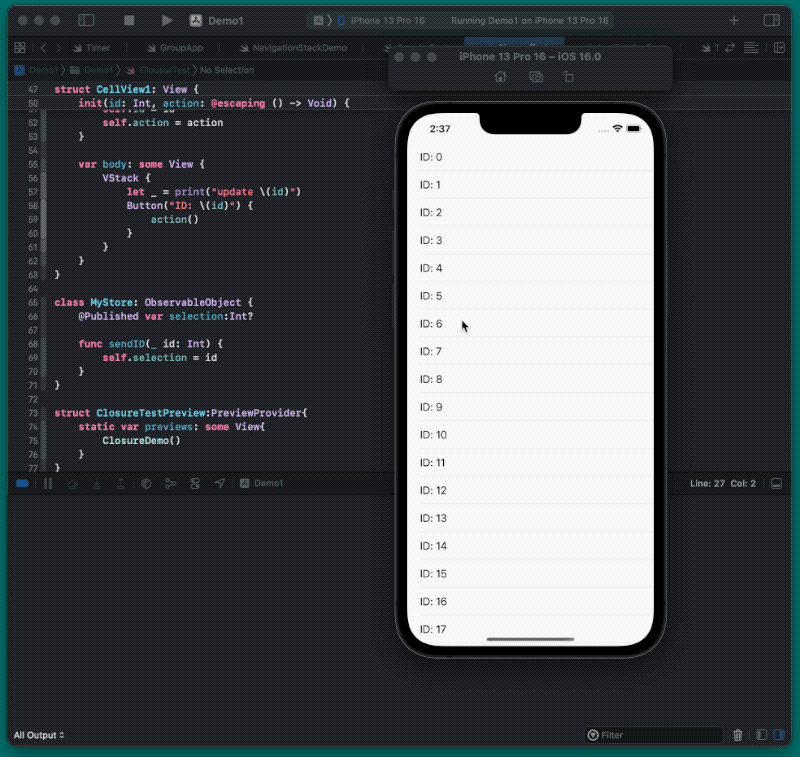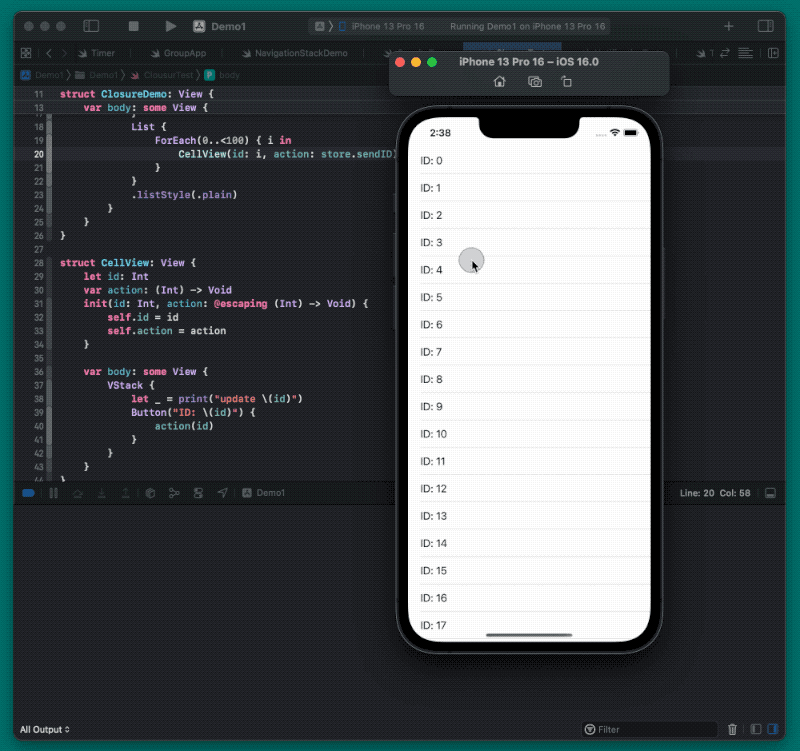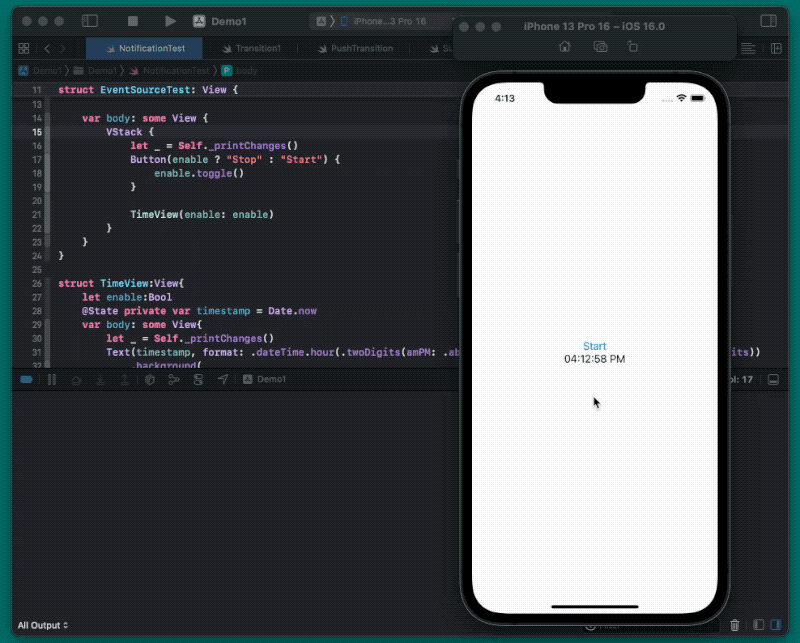# 避免 SwiftUI 视图的重复计算

## 视图状态的构成

• 使用 @State、@StateObject 这类属性包装器声明的变量
• 视图类型（ 符合 View 协议 ）的构造参数
• 例如 onReceive 这类的事件源

## 符合 DynamicProperty 协议的属性包装器

### DynamicProperty 的工作原理

``````public protocol DynamicProperty {
static func _makeProperty<V>(in buffer: inout _DynamicPropertyBuffer, container: _GraphValue<V>, fieldOffset: Int, inputs: inout _GraphInputs)
static var _propertyBehaviors: UInt32 { get }
mutating func update()
}
``````

``````@propertyWrapper public struct State<Value> : DynamicProperty {
internal var _value: Value
internal var _location: SwiftUI.AnyLocation<Value>? // SwiftUI 托管数据池中的数据引用
public init(wrappedValue value: Value)
public init(initialValue value: Value) {
_value = value // 创建实例时，只会暂存初始值
}
public var wrappedValue: Value {
get  //  guard let _location else { return _value} ...
nonmutating set // 只能改动 _location 指向的数据
}
public var projectedValue: SwiftUI.Binding<Value> {
get
}
// 在将视图加载到视图树中时，调用此方法，完成关联工作
public static func _makeProperty<V>(in buffer: inout _DynamicPropertyBuffer, container: _GraphValue<V>, fieldOffset: Int, inputs: inout _GraphInputs)
}
``````
• 在初始化 State 时，initialValue 仅被保存在 State 实例的内部属性 _value 中，此时，使用 Stae 包装的变量值没有被保存在 SwiftUI 的托管数据池中，并且 SwiftUI 也尚未在属性图中将其作为 Source of Truth 与视图关联起来。
• 当 SwiftUI 将视图加载到视图树时，通过调用 `_makeProperty` 完成将数据保存到托管数据池以及在属性图中创建关联的操作，并将数据在托管数据池中的引用保存在 `_location` （ AnyLocation 为引用类型，为 AnyLocationBase 的子类 ） 中。wrappedValue 的 get 和 set 方法都是针对 `_location` 操作的（ projectedValue 也一样 ）。
• 当 SwiftUI 将视图从视图树上删除时，会一并完成对 SwiftUI 数据池以及关联的清理工作。如此，使用 State 包装的变量，其存续期将与视图的存续期保持完全一致。并且 SwiftUI 会在其变化时自动更新（ 重新计算 ）对应的视图。

SwiftUI 上有一个困扰了不少人的问题：为什么无法在视图的构造函数中，更改 State 包装的变量值？了解了上述过程，问题便有了答案。

``````struct TestView: View {
@State private var number: Int = 10
init(number: Int) {
self.number = 11 // 更改无效
}
var body: some View {
Text("\(number)") // 首次运行，显示 10
}
}
``````

``````@propertyWrapper public struct StateObject<ObjectType> : DynamicProperty where ObjectType : ObservableObject {
internal enum Storage { // 通过内部定义的枚举来标注视图是否已经被加载、数据是否已被数据池托管
case initially(() -> ObjectType)
case object(ObservedObject<ObjectType>)
}

internal var storage: StateObject<ObjectType>.Storage
public init(wrappedValue thunk: @autoclosure @escaping () -> ObjectType) {
storage = .initially(thunk) // 初始化，视图尚未加载
}
@_Concurrency.MainActor(unsafe) public var wrappedValue: ObjectType {
get
}
@_Concurrency.MainActor(unsafe) public var projectedValue: SwiftUI.ObservedObject<ObjectType>.Wrapper {
get
}
// 在 DynamicProperty 要求的方法中，实现将实例保存在托管数据池，并将视图与托管实例的 objectWillChange 进行关联
public static func _makeProperty<V>(in buffer: inout _DynamicPropertyBuffer, container: _GraphValue<V>, fieldOffset: Int, inputs: inout _GraphInputs)
}
``````

@ObservedObject 与 @StateObject 最大的区别是，ObservedObject 并不会在 SwiftUI 托管数据池中保存引用对象的实例（ @StateObject 会将实例保存在托管数据池中 ），仅会在属性图中创建视图与视图类型实例中的引用对象的 objectWillChange 之间的关联。

``````@ObservedObject var store = Store() // 每次创建视图类型实例，都会重新创建 Store 实例
``````

### 避免非必要的声明

``````struct EnvObjectDemoView:View{
@EnvironmentObject var store:Store
var body: some View{
Text("abc")
}
}
``````

``````struct MyEnvKey: EnvironmentKey {
static var defaultValue = 10
}

extension EnvironmentValues {
var myValue: Int {
get { self[MyEnvKey.self] }
set { self[MyEnvKey.self] = newValue }
}
}

struct EnvDemo: View {
@State var i = 100
var body: some View {
VStack {
VStack {
EnvSubView()
}
.environment(\.myValue, i)
Button("change") {
i = Int.random(in: 0...100)
}
}
}
}

struct EnvSubView: View {
@Environment(\.myValue) var myValue // 声明了，但并没有在 body 中使用
var body: some View {
let _ = print("sub view update")
Text("Sub View")
}
}
``````

### 其他建议

• 需要跳跃视图层级时，考虑使用 Environment 或 EnvironmentObject
• 对于不紧密的 State 关系，考虑在同一个视图层级使用多个 EnvironmentObject 注入，将状态分离
• 在合适的场景中，可以使用 objectWillChange.send 替换 @Published
• 可以考虑使用第三方库，对状态进行切分，减少视图刷新几率
• 无需追求完全避免重复计算，应在依赖注入便利性、应用性能表现、测试难易度等方面取得平衡
• 不存在完美的解决方案，即使像 TCA 这类的热门项目，面对切分粒度高、层次多的 State 时，也会有明显的性能瓶颈

## 视图的构造参数

SwiftUI 会将视图类型的构造参数作为 Source of Truth 对待。与符合 DynamicProperty 协议的属性包装器主动驱动视图更新的机制不同，SwiftUI 在更新视图时，会通过检查子视图的实例是否发生变化（ 绝大多数都由构造参数值的变化导致 ）来决定对子视图更新与否。

``````struct SubView{
let name:String
let age:Int

var body: some View{
VStack{
Text(name)
Text("\(age)")
}
}
}

struct ContentView {
var body: some View{
SubView(name: "fat" , age: 99)
}
}
``````

### 简单、粗暴、高效的比对策略

• 创建新实例
• 将新实例与 SwiftUI 当前使用的实例进行比对
• 如实例发生变化，用新实例替换当前实例，对实例的 body 求值，并用新的视图值替换老的视图值
• 视图的存续期不会因为实体的更替有所改变

### 化整为零

``````struct Student {
var name: String
var age: Int
}

struct RootView:View{
@State var student = Student(name: "fat", age: 88)
var body: some View{
VStack{
StudentNameView(student: student)
StudentAgeView(student: student)
Button("random age"){
student.age = Int.random(in: 0...99)
}
}
}
}

// 分成小视图
struct StudentNameView:View{
let student:Student
var body: some View{
let _ = Self._printChanges()
Text(student.name)
}
}

struct StudentAgeView:View{
let student:Student
var body: some View{
let _ = Self._printChanges()
Text(student.age,format: .number)
}
}
``````

``````struct RootView:View{
@State var student = Student(name: "fat", age: 88)
var body: some View{
VStack{
StudentNameView(name: student.name) // 仅传递需要的数据
StudentAgeView(age:student.age)
Button("random age"){
student.age = Int.random(in: 0...99)
}
}
}
}

struct StudentNameView:View{
let name:String // 需要的数据
var body: some View{
let _ = Self._printChanges()
Text(name)
}
}

struct StudentAgeView:View{
let age:Int
var body: some View{
let _ = Self._printChanges()
Text(age,format: .number)
}
}
``````

### 让视图符合 Equatable 协议以自定义比对规则

• 让视图符合 Equatable 协议
• 为视图自定义判断相等的比对规则

``````struct RootView: View {
@State var student = Student(name: "fat", age: 88)
var body: some View {
VStack {
StudentNameView(student: student)
StudentAgeView(student: student)
Button("random age") {
student.age = Int.random(in: 0...99)
}
}
}
}

struct StudentNameView: View, Equatable {
let student: Student
var body: some View {
let _ = Self._printChanges()
Text(student.name)
}

static func == (lhs: Self, rhs: Self) -> Bool {
lhs.student.name == rhs.student.name
}
}

struct StudentAgeView: View, Equatable {
let student: Student
var body: some View {
let _ = Self._printChanges()
Text(student.age, format: .number)
}

static func== (lhs: Self, rhs: Self) -> Bool {
lhs.student.age == rhs.student.age
}
}
``````

### 闭包 —— 容易被忽略的突破点

``````struct ClosureDemo: View {
@StateObject var store = MyStore()
var body: some View {
VStack {
if let currentID = store.selection {
Text("Current ID: \(currentID)")
}
List {
ForEach(0..<100) { i in
CellView(id: i){ store.sendID(i) } // 使用尾随闭包的方式为子视图设定按钮动作
}
}
.listStyle(.plain)
}
}
}

struct CellView: View {
let id: Int
var action: () -> Void
init(id: Int, action: @escaping () -> Void) {
self.id = id
self.action = action
}

var body: some View {
VStack {
let _ = print("update \(id)")
Button("ID: \(id)") {
action()
}
}
}
}

class MyStore: ObservableObject {
@Published var selection:Int?

func sendID(_ id: Int) {
self.selection = id
}
}
````````````CellView(id: i){ store.sendID(i) }
``````

• 让 CellView 符合 Equatable 协议，不比较 action 参数
``````struct CellView: View, Equatable {
let id: Int
var action: () -> Void
init(id: Int, action: @escaping () -> Void) {
self.id = id
self.action = action
}

var body: some View {
VStack {
let _ = print("update \(id)")
Button("ID: \(id)") {
action()
}
}
}

static func == (lhs: Self, rhs: Self) -> Bool { // 将 action 排除在比较之外
lhs.id == rhs.id
}
}

ForEach(0..<100) { i in
CellView(id: i){ store.sendID(i) }
}
``````
• 修改构造参数中的函数定义，将 store 排除在 CellView 之外
``````struct CellView: View {
let id: Int
var action: (Int) -> Void // 修改函数定义
init(id: Int, action: @escaping (Int) -> Void) {
self.id = id
self.action = action
}

var body: some View {
VStack {
let _ = print("update \(id)")
Button("ID: \(id)") {
action(id)
}
}
}
}

ForEach(0..<100) { i in
CellView(id: i, action: store.sendID) // 直接传递 store 中的 sendID 方法，将 store 排除在外
}
``````## 事件源

• 控制生命周期

• 减小影响范围

``````struct EventSourceTest: View {
@State private var enable = false

var body: some View {
VStack {
let _ = Self._printChanges()
Button(enable ? "Stop" : "Start") {
enable.toggle()
}
TimeView(enable: enable) // 单独的视图，onReceive 只能导致 TimeView 被更新
}
}
}

struct TimeView:View{
let enable:Bool
@State private var timestamp = Date.now
var body: some View{
let _ = Self._printChanges()
Text(timestamp, format: .dateTime.hour(.twoDigits(amPM: .abbreviated)).minute(.twoDigits).second(.twoDigits))
.background(
Group {
if enable { // 只在需要使用时，才加载触发器
Color.clear
try? await Task.sleep(nanoseconds: 1000000000)
NotificationCenter.default.post(name: .test, object: Date())
}
}
if let date = notification.object as? Date {
timestamp = date
}
}
}
}
)
}
}

static let test = Notification.Name("test")
}
``````## 总结

 掌握 SwiftUI 的 task 修饰器 实时切换 Core Data 的云同步状态